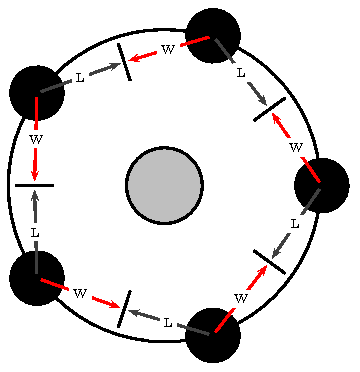So, despite the occasional mocking from my friends, I continue to write presentations in Latex using prosper and pstricks, hoping that when the time comes I'll be able to save the day. In this case I wanted to create images that exhibit the dining philosophers problem for a presentation about synchronization in multithreading environments.

Here's one that exhibits a deadlock: (L and W stands for Locked and Waiting respectively)And here's the Latex code:

\listfiles
\documentclass[a4paper]{article}

\usepackage{pstricks}
\usepackage{pst-node}
\usepackage{multido}
\usepackage{calc}

\begin{document}
\definecolor{DarkBlue1}{rgb}{.031,.251,.420}
\begin{pspicture}(12,10)
\psset{linewidth=2pt}

\pnode(6, 5){O} % center
\SpecialCoor
\pscircle[fillcolor=lightgray,fillstyle=solid](O){1}
\pscircle(O){4}

% philosophers and forks
\newcounter{degp}
\newcounter{degf}
\setcounter{degp}{0}
\setcounter{degf}{36}
\multido{\idx=0+1}{5}{
% Philosophers
\pscircle[fillcolor=black,fillstyle=solid]([nodesep=4,angle=\arabic{degp}]O){.7}
\pnode([nodesep=4, angle=\arabic{degp}]O){P\idx}
% Forks
\psline([nodesep=3.8, angle=\arabic{degf}]O)([nodesep=2.8, angle=\arabic{degf}]O)
\pnode([nodesep=3.3, angle=\arabic{degf}]O){F\idx}
}

\psset{nodesep=3pt, arrows=->}
\newcounter{next}
\newcounter{prev}
\setcounter{next}{0}
\setcounter{prev}{0}
\multido{\idx=0+1}{5}{
\setcounter{prev}{ (\idx + 4) - ((4 + \idx) / 5)*5}
% Locked (L) arrow
\ncline[linecolor=darkgray]{P\idx}{F\arabic{prev}}
\ncput*{L}
% Waiting (W) arrow
\ncline[linecolor=red]{P\idx}{F\idx}
\ncput*{W}
}
\end{pspicture}
\end{document}


The special coordinates mode is used (\SpecialCoor), which allows for defining placement by polar coordinates using a node (O in this case) as a reference. Additionally the calc package allows for counter commands that accept infix notation expressions instead of a simple value. (References:calc, pstricks)